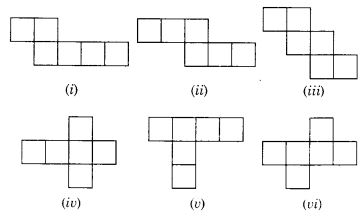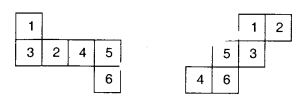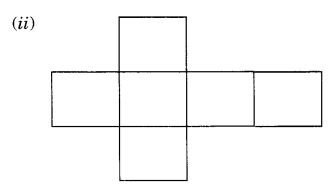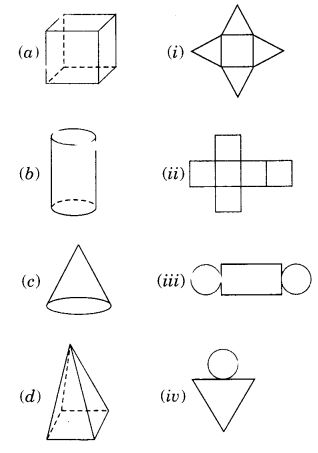NCERT Solutions for Class 7 Maths Chapter 15 Visualising Solid Shapes Ex 15.1 are part of NCERT Solutions for Class 7 Maths. Here we have given NCERT Solutions for Class 7 Maths Chapter 15 Visualising Solid Shapes Ex 15.1.

 Board CBSE Textbook NCERT Class Class 7 Subject Maths Chapter Chapter 15 Chapter Name Visualising Solid Shapes Exercise Ex 15.1 Number of Questions Solved 5 Category NCERT Solutions

## NCERT Solutions for Class 7 Maths Chapter 15 Visualising Solid Shapes Ex 15.1

Question 1.
Identify the nets which can be used to make cubes (cut out copies of the nets and try it):Solution:
(ii), (iii), (iv) and (vi).

Question 2.
Dice are cubes with dots on each face. Opposite faces of a die always have a total of seven dots on them. Here are two nets to make dice (cubes); the numbers inserted in each square indicate the number of dots in that box.Solution:
Insert suitable numbers in the blanks, remembering that the number on the opposite faces should total to 7.Question 3.Solution:
No, this cannot be a net for a die. Because one pair of opposite faces will have 1 and 4 on them and another pair of opposite faces will have 3 and 6 on them whose total is not equal to 7.

Question 4.
Here is an incomplete net for making a cube. Complete it in at least two different ways. Remember that a cube has six faces. How many are there on the net here? (Give two separate diagrams. If you like, you may use a squared sheet for easy manipulation).Solution:
In the net given here three faces are shown.Question 5.
Match the nets with appropriate solids:Solution:
(a) ↔ (ii)
(b) ↔ (iii)
(c) ↔ (iv)
(d) ↔ (i)

We hope the NCERT Solutions for Class 7 Maths Chapter 15 Visualising Solid Shapes Ex 15.1 helps you. If you have any query regarding NCERT Solutions for Class 7 Maths Chapter 15 Visualising Solid Shapes Ex 15.1, drop a comment below and we will get back to you at the earliest.